The X12 Procedure

TRANSFORM options ;

The TRANSFORM statement transforms or adjusts the series prior to estimating a regARIMA model. With this statement, the series can be Box-Cox (power) transformed. The "Prior Adjustment Factors" table is associated with the TRANSFORM statement.

Only one of the following options can appear in the TRANSFORM statement.

POWER=value

transforms the input series,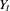, by using a Box-Cox power transformation,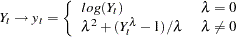The power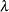must be specified (for example, POWER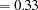). The default is no transformation (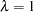); that is, POWER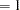. The log transformation (POWER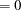), square root transformation (POWER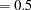), and the inverse transformation (POWER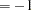) are equivalent to the corresponding FUNCTION= option.

 FUNCTION= Transformation Range forEquivalent Power Argument NONEall values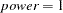LOG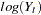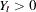for all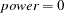SQRT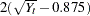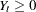for all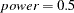INVERSE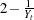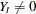for all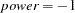LOGISTIC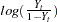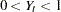for allnone equivalent
FUNCTION=NONE
FUNCTION=LOG
FUNCTION=SQRT
FUNCTION=INVERSE
FUNCTION=LOGISTIC
FUNCTION=AUTO
TYPE=NONE | LOG | SQRT | INVERSE | LOGISTIC | AUTO

the transformation to be applied to the series prior to estimating a regARIMA model. The transformation used by FUNCTION=NONE, LOG, SQRT, INVERSE, and LOGISTIC is related to the POWER= option as shown in Table 32.4. FUNCTION=AUTO uses selection based on Akaike’s information criterion (AIC) to decide between a log transformation and no transformation. The default is FUNCTION=NONE.

However, the FUNCTION= and POWER= options are not completely equivalent. In some cases, using the FUNCTION= option causes the program to automatically select other options. For instance, FUNCTION=NONE causes the default mode to be MODE=ADD in the X11 statement. Also, the choice of transformation invoked by the FUNCTION=AUTO option can impact the default mode of the X11 statement.

Note that there are restrictions on the value used in the POWER and FUNCTION options when preadjustment factors for seasonal adjustment are generated from a regARIMA model. When seasonal adjustment is requested with the X11 statement, any value of the POWER option can be used for the purpose of forecasting the series with a regARIMA model. However, this is not the case when factors generated from the regression coefficients are used to adjust either the original series or the final seasonally adjusted series. In this case, the only accepted transformations are the log transformation, which can be specified as POWER=0 (for multiplicative or log-additive seasonal adjustments) and no transformation, which can be specified as POWER=1 (for additive seasonal adjustments). If no seasonal adjustment is performed, any POWER transformation can be used. The preceding restrictions also apply to FUNCTION=NONE and FUNCTION=LOG.Previous Page | Next Page | Top of Page